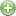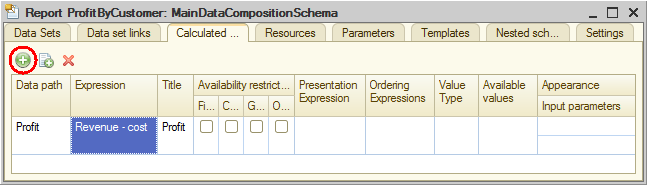# Calculated fields

This is the first time you need to display a field that is not available in the data set. Previously you always used fields defined in the data sets. To display the profit for rendered services by a customer, you need an additional field calculated as revenue minus service cost.

For this purpose the data composition system provides the option to define a calculated field.

Calculated fields are additional data composition schema fields whose values are calculated according to the specified formula.

1. Click the Calculated fields tab.
2. Click the Addbutton.

3. In the Data path column, type Profit.
4. In the Expression column, type the expression for calculating the field value (listing 13.14).

Listing 13.14. The expression for calculating the Profit field value
`Revenue - Cost`
The platform fills the calculated field title (which is displayed in the report) automatically, you have the option to change it (fig. 13.101).Fig. 13.101. Creating a calculated field

You can add a calculated field to report resources in order to calculate the group and overall totals by this field.

Next page: Resources

Be the first to know tips & tricks on business application development!